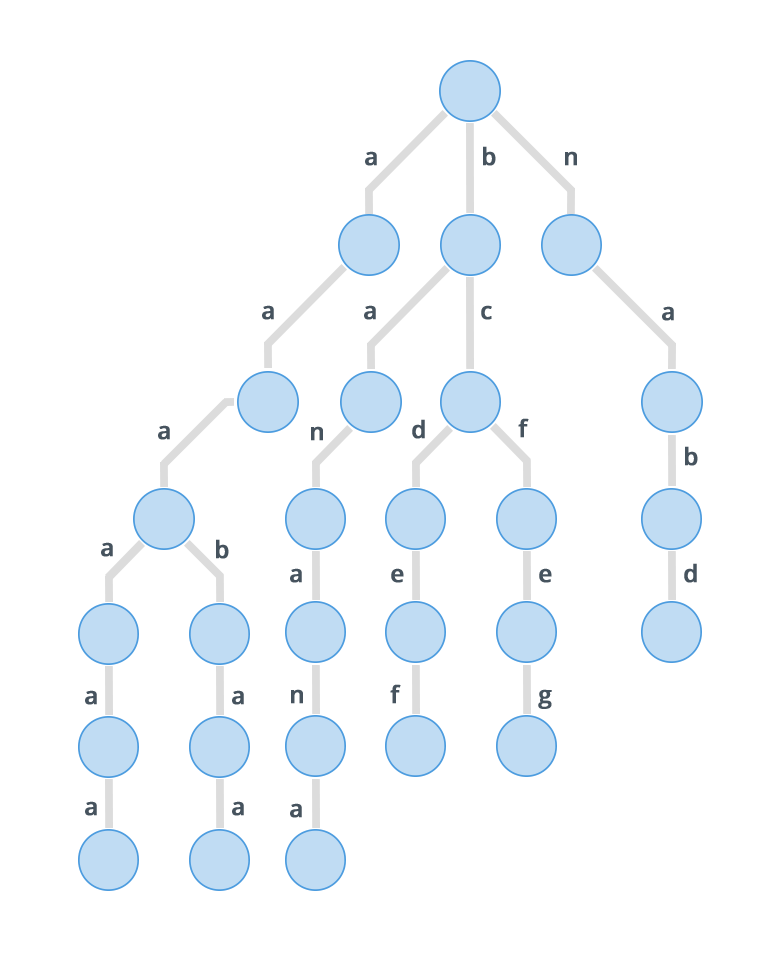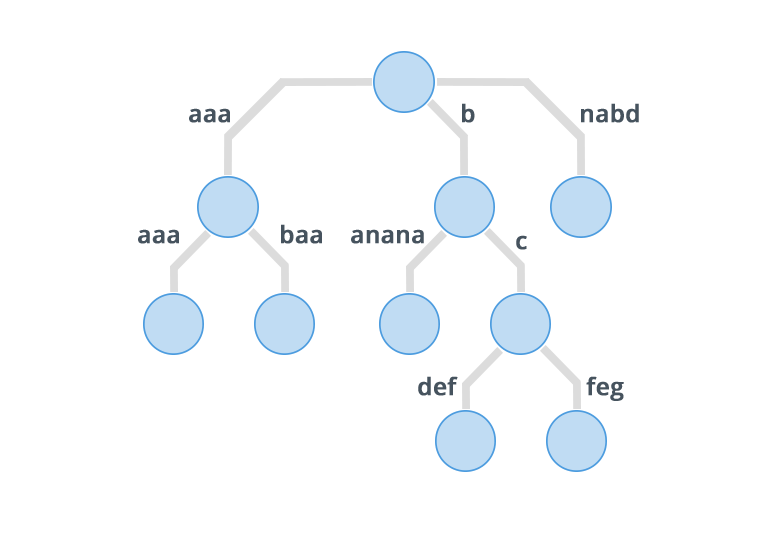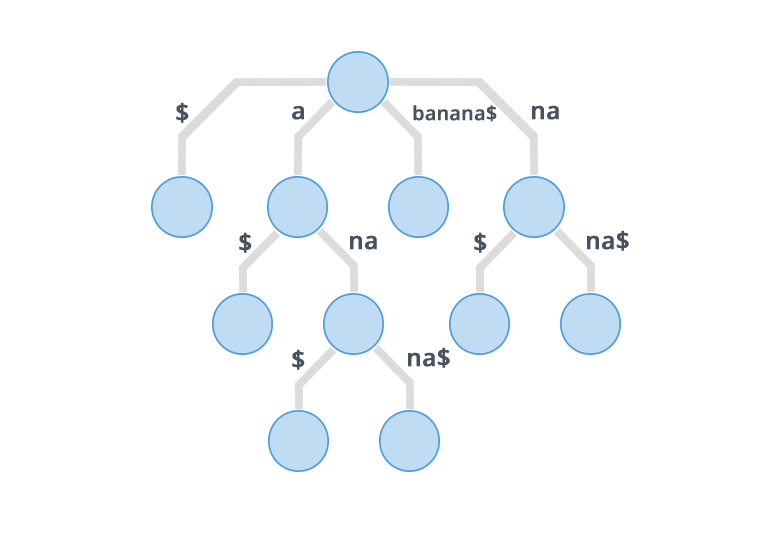Data Structures
Topics:
Suffix Trees
• Arrays
• Stacks
• Queues
• Hash Tables
• Trees
• Disjoint Data Structures

# Suffix Trees

Pre-requisite : Trie

Suffix tree is a compressed trie of all the suffixes of a given string. Suffix trees help in solving a lot of string related problems like pattern matching, finding distinct substrings in a given string, finding longest palindrome etc. In this tutorial following points will be covered:

• Compressed Trie
• Suffix Tree Construction (Brute Force)
• Brief description of Ukkonen's Algorithm

Before going to suffix tree, let's first try to understand what a compressed trie is.
Consider the following set of strings: {$"banana", "nabd", "bcdef", "bcfeg", "aaaaaa", "aaabaa"$ }
A standard trie for the above set of strings will look like:And a compressed trie for the given set of strings will look like:As it might be clear from the images show above, in a compressed trie, edges that direct to a node having single child are combined together to form a single edge and their edge labels are concatenated. So this means that each internal node in a compressed trie has atleast two children. Also it has atmost $N$ leaves, where $N$ is the number of strings inserted in the compressed trie. Now both the facts: Each internal node having atleast two children, and that there are $N$ leaves, implies that there are atmost $2N-1$ nodes in the trie. So the space complexity of a compressed trie is $O(N)$ as compared to the $O(N^2)$ of a normal trie.
So that is one reason why to use compressed tries over normal tries.

Before going to construction of suffix trees, there is one more thing that should be understood, Implicit Suffix Tree. In Implicit suffix trees, there are atmost $N$ leaves, while in normal one there should be exactly $N$ leaves. The reason for atmost $N$ leaves is one suffix being prefix of another suffix. Following example will make it clear. Consider the string $"banana"$
Implicit Suffix Tree for the above string is shown in image below:To avoid getting an Implicit Suffix Tree we append a special character that is not equal to any other character of the string. Suppose we append \$ to the given string then, so the new string is $"banana"$. Now its suffix tree will beNow let's go to the construction of the suffix trees.
Suffix tree as mentioned previously is a compressed trie of all the suffixes of a given string, so the brute force approach will be to consider all the suffixes of the given string as separate strings and insert them in the trie one by one. But time complexity of the brute force approach is $O(N^2)$, and that is of no use for large values of $N$.

The pseudo code for the brute force approach is given below:

function insert(T, string, start_index, length){
i = start_index
while(i < length)
if T.arr[string[i]]) is NULL
T.arr[string[i]] = new node()
T = T.arr[string[i]]
while(i < length)
T.label = T.label+string[i]
i = i+1
endwhile
return
endif

j=0, x=i
temp = T.arr[string[i]]
while j < temp.label.length and i < length && temp.label[j] = string[i]
i = i+1
j = j+1
endwhile

if i = length
return
endif

if j = temp.label.length
cnt = 0
for k = 'a' to 'z'
if temp.arr[k] = NULL
cnt = cnt+1
endif
endfor
if cnt = 27
while i < length
temp.label = temp.label + string[i]
i = i+1
endwhile
else
T = temp
endifelse
else
newInternalNode = new node()
k=0
while k < j
newInternalNode.label = newInternalNode.label + temp.label[k]
k = k+1
endwhile
newInternalNode.arr[string[j]] = new node()
while k < temp.label.length
newInternalNode.arr[string[j]].label+=temp.label[k]
k = k+1
endwhile
for k = 'a' to 'z'
newInternalNode.arr[string[j]].arr[k] = temp.arr[k]
endfor
T.arr[string[x]] = newInternalNode
T = T.arr[string[x]]
endifelse
endwhile
}

function makeSuffixTree(string, length){
Trie T
string = concatenate(string, "{")
for i = 0 to length
insertInTrie(T, string, i, length)
}


So as mentioned previously the above code will not be correct choice for large values of $N.$ Ukkonen's Algorithm comes to the rescue here.
Ukkonen's Algorithm constructs the suffix tree in a worst case time complexity of $O(N)$.

Ukkonen's Algorithm divides the process of constructing suffix tree into phases and each phase is further divided into extensions. In $i^{th}$ phase $i^{th}$ character is introduced in the trie. In $i^{th}$ phase, all the suffixes of the string $S[1..i]$ are inserted into the trie, and inserting $j^{th}$ suffix in a phase is called $j^{th}$ extension of that phase. So, in $i^{th}$ phase there are $i$ extensions and overall there $N$ such phases, where $N$ is the length of given string. Right now it must look like a $O(N^2)$ task, but the algorithm exploits the fact that these are suffixes of same string and introduces several tricks that bring down the time complexity to $O(N)$.

Let's see how to perform the $j^{th}$ extension of $i^{th}$ phase. In $j^{th}$ extension of $i^{th}$ phase string $S[j...i]$ is to be inserted. Before going for phase $i$, $i-1$ phases are already complete, that means we have a trie having all suffixes of string $S[1...i-1]$. So search for the path of string $S[j..i-1]$ in the trie. Now there are 3 possibilities and each of those correspond to one rule, that has to be followed.

1. The complete string is found and path ends at a leaf node. In that case the $i^{th}$ character is appended to last edge label and no new node is created.
2. The complete string is found and path ends in between an edge label, and the next character of edge label is not equal to $S[i]$ or the path ends at an internal node. In this case new nodes are created. If the path ends in an internal node then a new leaf node is created, and if the path ends in between an edge label then a new internal node and one new leaf node is created.
3. Complete suffix $S[j..i-1]$ is found and the path ended in between an edge label and the next character of that edge label is equal to $i^{th}$ character. In that case do nothing.

So given above are the 3 extension rules used to perform extensions in a phase.
Note that still we are doing $N$ phases and in $i^{th}$ phase we are performing $i$ extensions.
For every extension we need to find the path of a string $S[j...i]$ in the trie built in previous phases. If we go with brute force approach time complexity will be $O(N^3)$, for that we use suffix links, which are explained below:

Suffix Links: Suppose a string $X$ is present in the trie, and its path from root ends at a node $v$, and string $aX$ is also present in the trie where $a$ is any character, and its path from root ends at a node $w$, then a link from $w$ to $v$ is called a Suffix Link.

Now how does a suffix link help? When we have to perform $j^{th}$ extension of phase $i$, we have to look for end of path of string $S[j...i-1]$, and in the next phase look for end of path of string $S[j+1...i-1]$, but before coming to phase $i$, we have performed $i-1$ phases, that means we have inserted strings $S[j..i-1]$ and $S[j+1...i-1]$ in the trie. Now clearly $S[j...i-1]$ is nothing but $S[j]S[j+1...i-1]$, so we will have a suffix link from node ending at path $S[j..i-1]$ to node ending at path $S[j+1...i-1]$, so instead of traversing down from root for $(j+1)^{th}$ extension of $i^{th}$ phase, we can make use of the suffix link.
Use of suffix link makes processing of a phase an $O(number of nodes)$ process, and number of nodes in a compressed trie are of $O(N)$. So right now each phase is done in $O(N)$ and there are $N$ such phases, so overall complexity right now is $O(N^2)$.

Before going further there is one more problem, and that is the edge labels. If the edge labels are stored as strings space complexity will turn out to be $O(N^2)$, no matter the what the number of nodes are. So for that instead of using strings as edge label, use two variables for each label and those will denote the start index and end index of the label in the string. That way each label will take constant space and overall space complexity will be $O(N)$.

There are several more tricks that help in bringing down the complexity to linear.

In any phase, the extension rules are applied in the order. In first few extensions, rule 1 is applied, in the next few extensions rule 2 is applied and in the rest rule 3 is applied.

If in $i^{th}$ phase rule 3 is applied in extension $j$ for the first time, then in all the extensions after that i.e. in extensions $j+1$ to $i$, rule 3 will be applied, so its ok to halt a phase as soon as rule 3 starts applying.

Once a leaf node is created it will always remain a leaf node, only edge label of the edge between itself and its parent, will keep on increasing because of application of rule 1, and also for all the leaf node the end index (discussed earlier) will remain same, so in any phase rule 1 can also be applied in a constant time by maintain a global end index for all the leaf nodes.

New leaf nodes are created when rule 2 is applied, and in all the extensions in which rule 2 is applied in any phase $i-1$, in the next phase $i$, rule 1 will be applied in all those extensions.

So a maximum of $N$ times rule 2 will be applied as there are $N$ leaves, so this means all the phases can be completed in complexity $O(N)$.

Contributed by: Vaibhav Jaimini